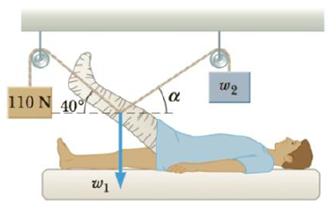Chapter 4, Problem 40P

Chapter
Section
Textbook Problem

The leg and cast in Figure P4.40 weigh 220 N (w1). Determine the weight w2 and the angle α needed so that no force is exerted on the hip joint by the leg plus the cast.Figure P4.40

To determine
The weight ( w2 ) and angle ( α ).

Explanation

Section 1:

To determine: The angle ( α ).

Answer: The angle ( α ) is 60.56° .

Explanation:

Given Info: Weight of the leg and cast ( w1 ) is 220 N.

The free body diagram is given below.

From the above diagram,

(110N)cos40ο=w2cosα                              (I)

w2sinα+(110N)sin40°=w1                         (II)

• w1 and w2 are the weights.
• α is the angle at which w2 is oriented with respect to the horizon.

From Equation (I),

w2=w1(110N)sin40οsinα                                             (III)

From Equation (I) and (II),

α=tan1(w1(110N)sin40°(110N)cos40°)                                  (IV)

Substitute 110 N for w1 in Equation (V) to get α

Still sussing out bartleby?

Check out a sample textbook solution.

See a sample solution

The Solution to Your Study Problems

Bartleby provides explanations to thousands of textbook problems written by our experts, many with advanced degrees!

Get Started